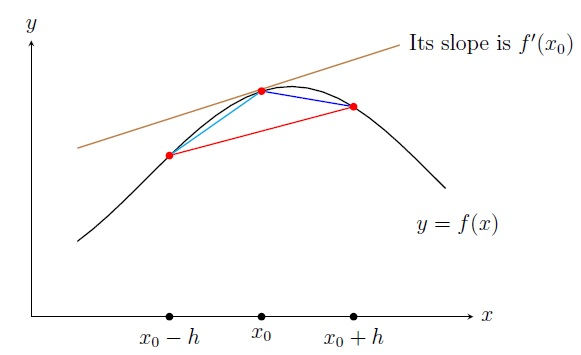Numerical Analysis Home

## Numerical Differentiation

In this section we will numerically find $$f'(x)$$ evaluated at $$x=x_0$$. We need numerical techniques for derivatives when $$f'(x)$$ has a complicated expression or $$f(x)$$ is not explicitly given. By the limit definition of derivative, $f'(x_0)=\lim_{h\to 0}\frac{f(x_0+h)-f(x_0)}{h}.$ So when $$h>0$$ is small, we have $f'(x_0)\approx \frac{f(x_0+h)-f(x_0)}{h},$ which is called the two-point forward difference formula (FDF). Similarly the two-point backward difference formula (BDF) is $f'(x_0)\approx \frac{f(x_0)-f(x_0-h)}{h}.$ Taking the average of the FDF and BDF, we get the two-point centered difference formula (CDF) is $f'(x_0)\approx \frac{f(x_0+h)-f(x_0-h)}{2h}.$Note that CDF gives better accuracy than FDF and BDF (explained later). But CDF does not work if $$f(x)$$ is not known in one side of $$x_0$$. All the difference formulas suffer from round-off errors when $$h$$ is too small.

Example. $$f(x)=x^2e^{x}$$. Approximate $$f'(1)$$ using the FDF, BDF, and CDF with $$h=0.2$$.

Solution. \begin{align*} \text{Two-point FDF}:\; f'(1) &\approx \frac{f(1+0.2)-f(1)}{0.2}=\frac{4.78-2.71}{0.2}=10.35 \\ \text{Two-point BDF}:\; f'(1) &\approx \frac{f(1)-f(1-0.2)}{0.2}=\frac{2.71-1.42}{0.2}=6.45\\ \text{Two-point CDF}:\; f'(1) &\approx \frac{f(1+0.2)-f(1-0.2)}{2(0.2)}= \frac{4.78-1.42}{0.4}=8.4 \end{align*} Analytically we know $$f'(1)=3e$$. So the absolute errors are $$|10.35-3e|=2.19$$, $$|6.45-3e|=1.7$$, and $$|8.4-3e|=0.24$$ respectively. So CDF gives the least error.

Errors in finite difference formulas: The maximum error in FDF is $\frac{h}{2} \displaystyle\max_{x\in (x_0,x_0+h)}|f''(x)|.$

By the Taylor's theorem on $$f$$ about $$x_0$$, we get \begin{align*} f(x)&= f(x_0)+\frac{f'(x_0)}{1!}(x-x_0)+ \frac{f''(\xi)}{2!}(x-x_0)^2. \end{align*} Plugging $$x=x_0+h$$, we get \begin{align*} f(x_0+h)&= f(x_0)+\frac{f'(x_0)}{1!}h+ \frac{f''(\xi_1)}{2!}h^2, \end{align*} for some $$\xi_1\in (x_0,x_0+h)$$. Solving for $$f'(x_0)$$, we get $f'(x_0)= \frac{f(x_0+h)-f(x_0)}{h}-\frac{f''(\xi_1)}{2}h.$

So the error in FDF is $$O(h)$$ (i.e., absolute error $$\leq ch$$ for some $$c>0$$). It means small step size $$h$$ results in more accurate derivative. We say FDF is first-order accurate. Similarly BDF is also first-order accurate with the maximum error $\frac{h}{2} \displaystyle\max_{x\in (x_0-h,x_0)}|f''(x)|.$ The maximum error in CDF is $\frac{h^2}{6} \displaystyle\max_{x\in (x_0-h,x_0+h)}|f'''(x)|.$ So the error in CDF is $$O(h^2)$$, i.e., CDF is second-order accurate which is better than first-order accurate as $$h^2<< h$$ for small $$h >0$$.

For CDF, note that \begin{align*} f(x_0+h)&= f(x_0)+\frac{f'(x_0)}{1!}h+ \frac{f''(x_0)}{2!}h^2+\frac{f'''(\xi_1)}{3!}h^3,\\ f(x_0-h)&= f(x_0)-\frac{f'(x_0)}{1!}h+ \frac{f''(x_0)}{2!}h^2-\frac{f'''(\xi_2)}{3!}h^3, \end{align*} for some $$\xi_1\in (x_0,x_0+h)$$ and $$\xi_2\in (x_0-h,x_0)$$. Subtracting we get, \begin{align*} f(x_0+h)-f(x_0-h)&= 2f'(x_0)h+\frac{f'''(\xi_1)+f'''(\xi_2)}{6}h^3\\ \frac{f(x_0+h)-f(x_0-h)}{2h}&= f'(x_0)+\frac{f'''(\xi_1)+f'''(\xi_2)}{12}h^2\\ f'(x_0) &= \frac{f(x_0+h)-f(x_0-h)}{2h}-\frac{f'''(\xi_1)+f'''(\xi_2)}{12}h^2. \end{align*} Assuming continuity of $$f'''$$ and using the IVT on $$f'''$$, we get $f'''(\xi)=\frac{f'''(\xi_1)+f'''(\xi_2)}{2},$ for some $$\xi\in (\xi_2,\xi_1)\subset (x_0-h,x_0+h)$$. Thus $f'(x_0)= \frac{f(x_0+h)-f(x_0-h)}{2h}-\frac{f'''(\xi)}{6}h^2.$

Example. Consider $$f(x)=x^2e^{x}$$. Find the maximum error in approximating $$f'(1)$$ by the FDF, BDF, and CDF with $$h=0.2$$.

Solution. $$f''(x)=(x^2+4x+2)e^{x}$$ and $$f'''(x)=(x^2+6x+6)e^{x}$$ are increasing functions for $$x>0$$. So $$\max_{x\in (1,1.2)}|f''(x)|=|f''(1.2)|=27.3$$. \begin{align*} \text{Maximum error in two-point FDF}:\;&\frac{0.2}{2}\max_{x\in (1,1.2)}|f''(x)|=0.1|f''(1.2)|=2.73 \\ \text{Maximum error in two-point BDF}:\;& \frac{0.2}{2}\max_{x\in (0.8,1)}|f''(x)|=0.1|f''(1)|=1.9\\ \text{Maximum error in two-point CDF}:\;& \frac{(0.2)^2}{6}\max_{x\in (0.8,1.2)}|f'''(x)|=\frac{0.04}{6}|f'''(1.2)|=0.32\\ \end{align*}

Derivative from Lagrange polynomial: If $$f$$ is not explicitly given but we know $$(x_i,f(x_i))$$ for $$i=0,1,\ldots,n$$, then $$f$$ is approximated by the Lagrange polynomial: $f(x)=\sum_{i=0}^n f(x_i)L_i(x)+\frac{f^{(n+1)}(\xi(x))}{(n+1)!} \prod_{i=0}^n (x-x_i),$ where $$\xi\in (x_0,x_n)$$ and $$L_i(x)=\displaystyle\prod_{\substack{j=0\\j\neq i}}^n\frac{(x-x_j)}{(x_i-x_j)}$$. Differentiating both sides and evaluating at $$x=x_j$$, we get (steps skipped but note that $$\frac{d}{dx} \prod_{i=0}^n (x-x_i)\Big]_{x=x_j}=\prod_{\substack{i=0\\i\neq j}}^n (x_j-x_i)$$) $f'(x_j)=\sum_{i=0}^n f(x_i)L_i'(x_j)+\frac{f^{(n+1)}(\xi)}{(n+1)!} \prod_{\substack{i=0\\i\neq j}}^n (x_j-x_i).$ If the points $$x_0,x_1,\ldots,x_n$$ are equally-spaced, i.e., $$x_j=x_0+jh$$, then we get $f'(x_j)=\sum_{i=0}^n f(x_i)L_i'(x_j)+\frac{f^{(n+1)}(\xi)}{(n+1)!} O(h^n).$ It can be verified that two-point FDF and BDF are obtained from the preceding equation using $$n=1$$. Similarly $$n=2$$ gives the three-point FDF and BDF and two-point CDF whose errors are $$O(h^2)$$: \begin{align*} \text{Three-point FDF}:\; f'(x_0) &\approx\frac{-3f(x_0)+4f(x_0+h)-f(x_0+2h)}{2h}\\ \text{Three-point BDF}:\; f'(x_0) &\approx\frac{3f(x_0)-4f(x_0-h)+f(x_0-2h)}{2h} \\ \end{align*}

Example. From the following table approximate $$f'(1)$$ by the three-point FDF and BDF. $\begin{array}{|c|c|c|c|c|c|} \hline x & 0.6 & 0.8 & 1 & 1.2 & 1.4\\ \hline f(x) & 0.65 & 1.42 & 2.71 & 4.78 & 7.94\\ \hline \end{array}$ Solution. Here $$h=0.2$$. \begin{align*} \text{Three-point FDF}:\; f'(1) &\approx\frac{-3f(1)+4f(1+0.2)-f(1+2(0.2))}{2(0.2)}=7.62\\ \text{Three-point BDF}:\; f'(1) &\approx\frac{3f(1)-4f(1-0.2)+f(1-2(0.2))}{2(0.2)} =7.75 \end{align*} Note that the table is given for $$f(x)=x^2e^x$$. So $$f'(1)=3e$$. Then the absolute errors are $$|7.62-3e|=0.53$$ and $$|7.75-3e|=0.4$$ respectively. Notice that three-point FDF and BDF give less error than two-point FDF and BDF respectively.

Last edited